## Thomson Scattering

If the energy of the scattered photon is much bigger than the binding energy of the atom,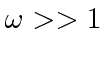eV. then cross section approaches that for scattering from a free electron, Thomson Scattering. We still neglect the effect of electron recoil so we should also require that. Start from the Kramers-Heisenberg formula.Thedenominators are much larger thanwhich is of the order of the electron's kinetic energy, so we can ignore the second two terms. (Even if the intermediate and final states have unbound electrons, the initial state wave function will keep these terms small.)This scattering cross section is of the order of the classical radius of the electron squared, and is independent of the frequency of the light.

The only dependence is on polarization. This is a good time to take a look at the meaning of the polarization vectors we've been carrying around in the calculation and at the lack of any wave-vectors for the initial and final state. A look back at the calculation shows that we calculated the transition rate from a state with one photon with wave-vectorand polarizationto a final state with polarization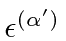. We have integrated over the final state wave vector magnitude, subject to the delta function giving energy conservation, but, we have not integrated over final state photon direction yet, as indicated by the. There is no explicit angular dependence but there is some hidden in the dot product between initial and final polarization vectors, both of which must be transverse to the direction of propagation. We are ready to compute four different differential cross sections corresponding to two initial polarizations times two final state photon polarizations. Alternatively, we average and/or sum, if we so choose.

In the high energy approximation we have made, there is no dependence on the state of the atoms, so we are free to choose our coordinate system any way we want. Set the z-axis to be along the direction of the initial photon and set the x-axis so that the scattered photon is in the x-z plane (). The scattered photon is at an angleto the initial photon direction and at. A reasonable set of initial state polarization vectors isPickto be in the scattering plane (x-z) defined as the plane containing bothandand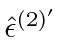to be perpendicular to the scattering plane.is then at an angleto the x-axis.is along the y-axis. We can compute all the dot products.From these, we can compute any cross section we want. For example, averaging over initial state polarization and summing over final is just half the sum of the squares of the above.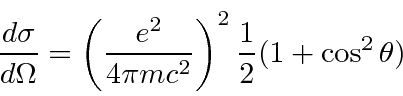Even if the initial state is unpolarized, the final state can be polarized. For example, for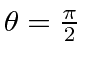, all of the above dot products are zero except. That means only the initial photons polarized along the y direction will scatter and that the scattered photon is 100% polarized transverse to the scattering plane(really just the same polarization as the initial state). The angular distribution could also be used to deduce the polarization of the initial state if a large ensemble of initial state photons were available.

For a definite initial state polarization (at an angleto the scattering plane, the component alongisand alongis. If we don't observe final state polarization we sumand have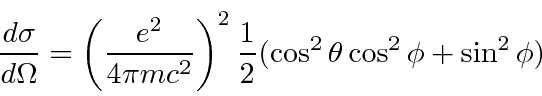For atoms with more than one electron, this cross section will grow as.

Jim Branson 2013-04-22